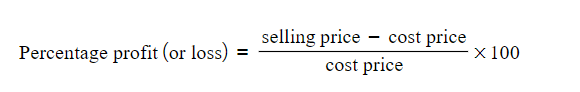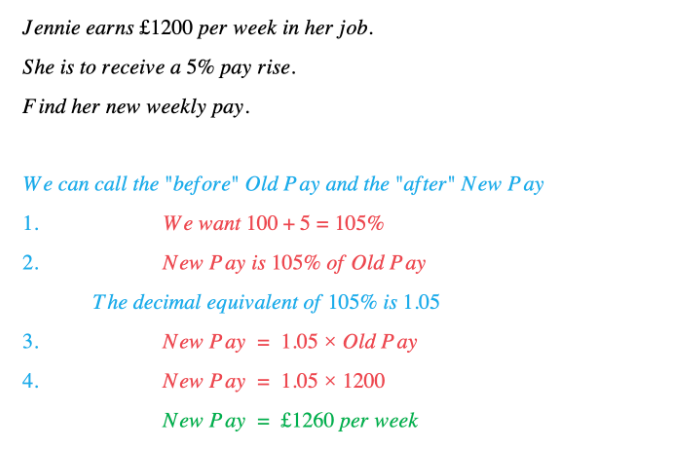# Edexcel IGCSE Maths复习笔记 1.7.2 Percentage Increases & Decreases

Edexcel IGCSE Maths复习笔记 1.7.2 Percentage Increases & Decreases

#### How do I increase or decrease by a percentage?

• Identify “before” & “after” quantities
• Find percentage of “before” that we want:
• Increase – add percentage to 100
• Decrease – subtract percentage from 100

• Write down a statement connecting “before” and “after”:
• “after is a percentage of before”

• Write down the statement as an equation using decimal equivalent
• remember “is” means “=”

• Substitute and solve

#### How do I find percentage profit (or loss)?

• Calculate percentage profit (or loss)• You can identify whether there is a profit or loss
• cost price < selling price = profit (positive)
• cost price > selling price = loss (negative)

Worked Example